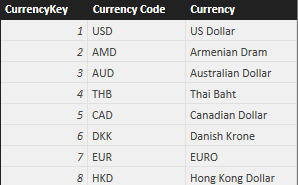DAX has an internal tracking of physical columns (lineage) that keeps references to original columns after certain transformations applied in a DAX expression. In this puzzle, you have to predict the result of two similar DAX queries.

## Scenario

The data model has a simple Currency table with three columns: CurrencyKey, Currency Code, and Currency.## The Challenge

Consider the following DAX table expression (we will reference it as Q1):

```CALCULATETABLE (
Currency,
FILTER (
CROSSJOIN (
VALUES ( Currency[Currency Code] ),
SELECTCOLUMNS (
VALUES ( Currency[Currency Code] ),
"Another", Currency[Currency Code]
)
),
[another] <> Currency[Currency Code]
)
)
```

If you create a calculated table based on such expression, what is the result?

• An error
• An empty table
• A table that has all the rows of the original Currency table

You can download the sample file and execute the query, but in this case we suggest you to not do that until you formulated your answer (and explanation).

Before looking at the solution, try to predict the result of this slightly different query (we will reference it as Q2; it is similar, but not identical, to Q1):

```CALCULATETABLE (
Currency,
FILTER (
CROSSJOIN (
VALUES ( Currency[Currency Code] ),
SELECTCOLUMNS (
VALUES ( Currency[Currency Code] ),
"Another", Currency[Currency Code] & ""
)
),
[another] <> Currency[Currency Code]
)
)
```

Again, what is the result?

• An error
• An empty table
• A table that has all the rows of the original Currency table

Once you formulated your answers, you can try the expressions in the sample file and view the solution. If the result of the query is not what you would have expected, spend some time trying to figure out why it was different.

## Hints

The puzzle is all about your understanding of data lineage and filter context in DAX. Moreover, think carefully about the result of each function included in the expression.

## Solution

To see the solution, just press the button below.